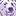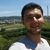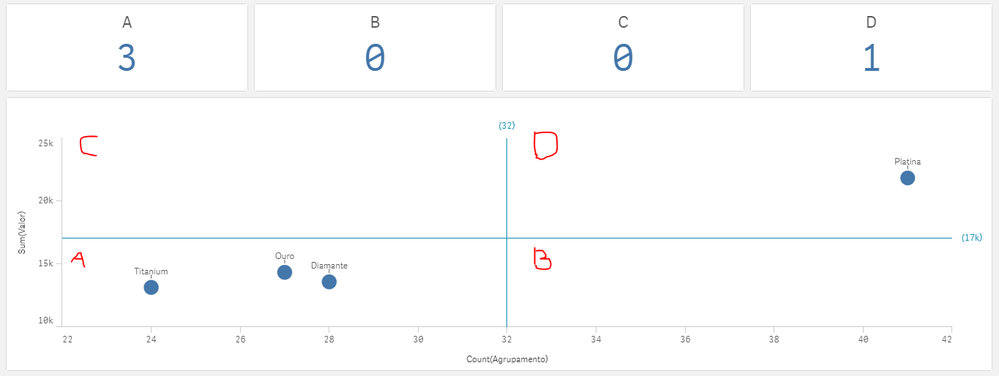# New to Qlik Sense

If you’re new to Qlik Sense, start with this Discussion Board and get up-to-speed quickly.

cancel
Showing results for
Did you mean:Creator

## Count of items lying in each quadrant in a scatter plot

Hi Guys,

I have created a scatter plot based on Stores and the sales by the each store. I would like to know the count of stores that lie in each quadrant. Is this possible? Please let me know.

Best Regards,

Rony

Labels (4)

• ### SaaS

1 Solution

Accepted SolutionsCreator

Okay my bad again, in my original reply I had the \$(= but they shouldn't be there so you can remove them

``````Count({<Location=
{"=((Sum({\$<Year={'2019'}>}Value)/Sum({\$<Year={'2019'}>}Volume))/(Sum({\$<Year={'2018'}>}Value)/Sum({\$<Year={'2018'}>}Volume))-1)<=0"}
>*<Location=
{"=(Sum({\$<Year={'2019'}>}Volume)/Sum({\$<Year={'2018'}>}Volume)-1)<=0"}>}
distinct Location)``````

How is this?

17 RepliesCreator II

Hi

I suppose you put right reference lines

you can use set analysis filters on measures``````A
Count({<Agrupamento={"=sum(Valor)<=17000"}>*<Agrupamento={"=count(Valor)<=32"}>} distinct Agrupamento)

B
Count({<Agrupamento={"=sum(Valor)<=17000"}>*<Agrupamento={"=count(Valor)>32"}>} distinct Agrupamento)

C
Count({<Agrupamento={"=sum(Valor)>17000"}>*<Agrupamento={"=count(Valor)<=32"}>} distinct Agrupamento)

D
Count({<Agrupamento={"=sum(Valor)>17000"}>*<Agrupamento={"=count(Valor)>32"}>} distinct Agrupamento)``````

RegardsCreator
Author

Hi Yoshida,

What do you mean by Agrupamento? Could you explain this expression to me?

Best Regards,

RonyCreator

Could you post a screenshot of your scatter graph?

What are the axes? Sales and what?Creator II

Hi,

In fact, I had forgotten to translate the formula,

Agrupamento would be your Stores

Valor would be the sales or whatever measure you want to put in the chart

RegardsCreator
Author

Yes. X axis is the growth in Average Selling Price and Y axis is the Volume growth. Attached is the screenshot.Creator

Okay, then use Yoshidaqlik's solution but change the values to your corresponding values

``Count({<Store={"=avg(sellingprice)<=0"}>*<Store={"=avg(volumegrowth)<=0"}>} distinct Store)``

I don't know what expressions you used to calculate the selling prices and volume growths, but put them in the above expressions.Creator
Author

ok. Thanks Anseg and Yoshida. I'll try this and get back.

RonyCreator
Author

Hi Guys,

The horizontal line represents ASP growth and vertical line represent Volume growth. I have taken store name as dimension. Formula for ASP growth used is, ((Sum({\$<Year={"2019"}>}ASP))/(Sum({\$<Year={"2018"}>}ASP)))-1 and volume growth is ((Sum({\$<Year={"2019"}>}Volume))/Sum({\$<Year={"2018"}>}Volume)))-1. Please guide me with respect to the complete expression.

Best Regards,

RonyCreator

Hi,

Does this work?

``````Count({<Store=
{"=\$(((avg({\$<Year={'2019'}>}ASP))/(avg({\$<Year={'2018'}>}ASP)))-1)<=0"}
>*<Store=
{"=\$(((avg({\$<Year={'2019'}>}Volume))/(avg({\$<Year={'2018'}>}Volume)))-1)<=0"}
distinct Store)``````Tags
Community Browser